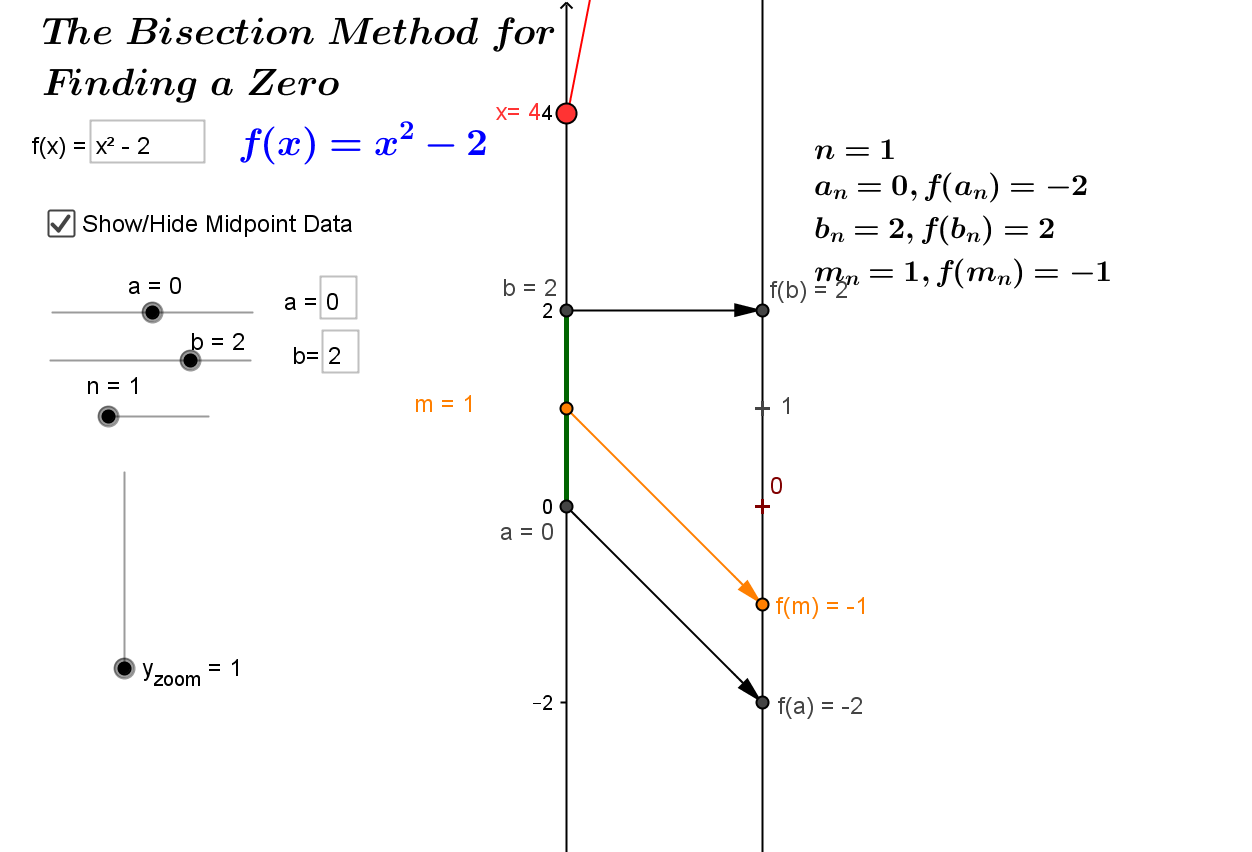MAA Minicourse #7 ¤
January 7 and 9, 2016
Making Sense of Calculus with Mapping Diagrams
Part I
Linearity, Limits, Derivatives, and Differential Equations

Martin Flashman
Professor of Mathematics
Humboldt State University

Abstract:
In this mini-course participants will learn how to use mapping diagrams (MD) to visualize functions for many calculus concepts. For a given function, f, a mapping diagram is basically a visualization of a function table that can be made dynamic with current technology. The MD represents  x  and f(x) from the table as points on parallel axes and arrows between the points indicate the function relation. The course will start with an overview of MD’s and then connect MD's to key calculus definitions and theory including: linearity, limits, derivatives, integrals, and series. Participants will learn how to use MD’s to visualize concepts, results and proofs not easily realized with graphs for both single and multi-variable calculus. Participants are encouraged to bring a laptop with wireless capability.

1.Mapping Diagrams. ¤

1.1 What is a mapping diagram?
Introduction and simple examples from the past: Napiers LogarithmSee: http://www.maa.org/press/periodicals/convergence/logarithms-the-early-history-of-a-familiar-function-john-napier-introduces-logarithms

Logarithms: The Early History of a Familiar Function - John Napier Introduces Logarithms  by Kathleen M. Clark (The Florida State University) and Clemency Montelle (University of Canterbury)1.2 Functions: Tables, Mapping Diagrams, and Graphs [Worksheet 1, 2 and 3.]
Understanding functions using tables. mapping diagrams, and graphs.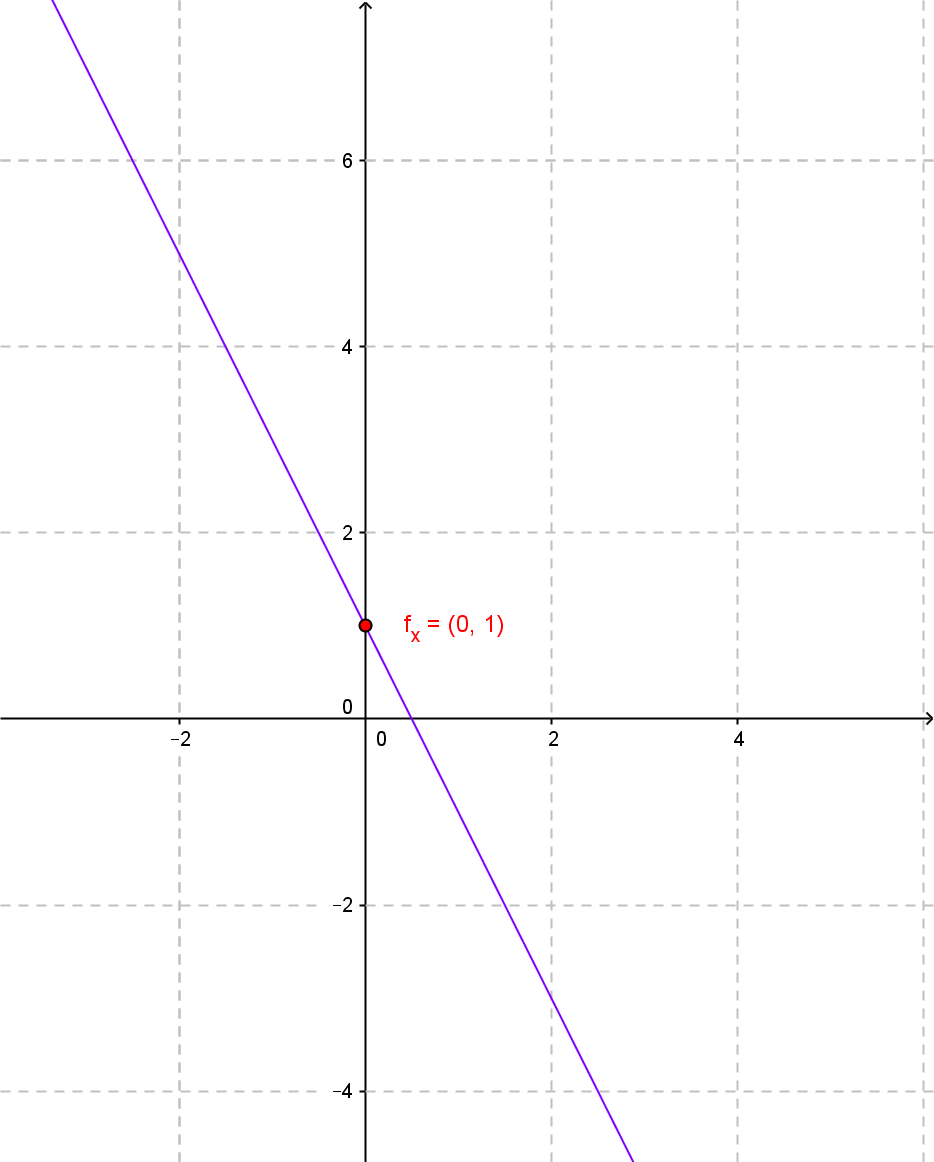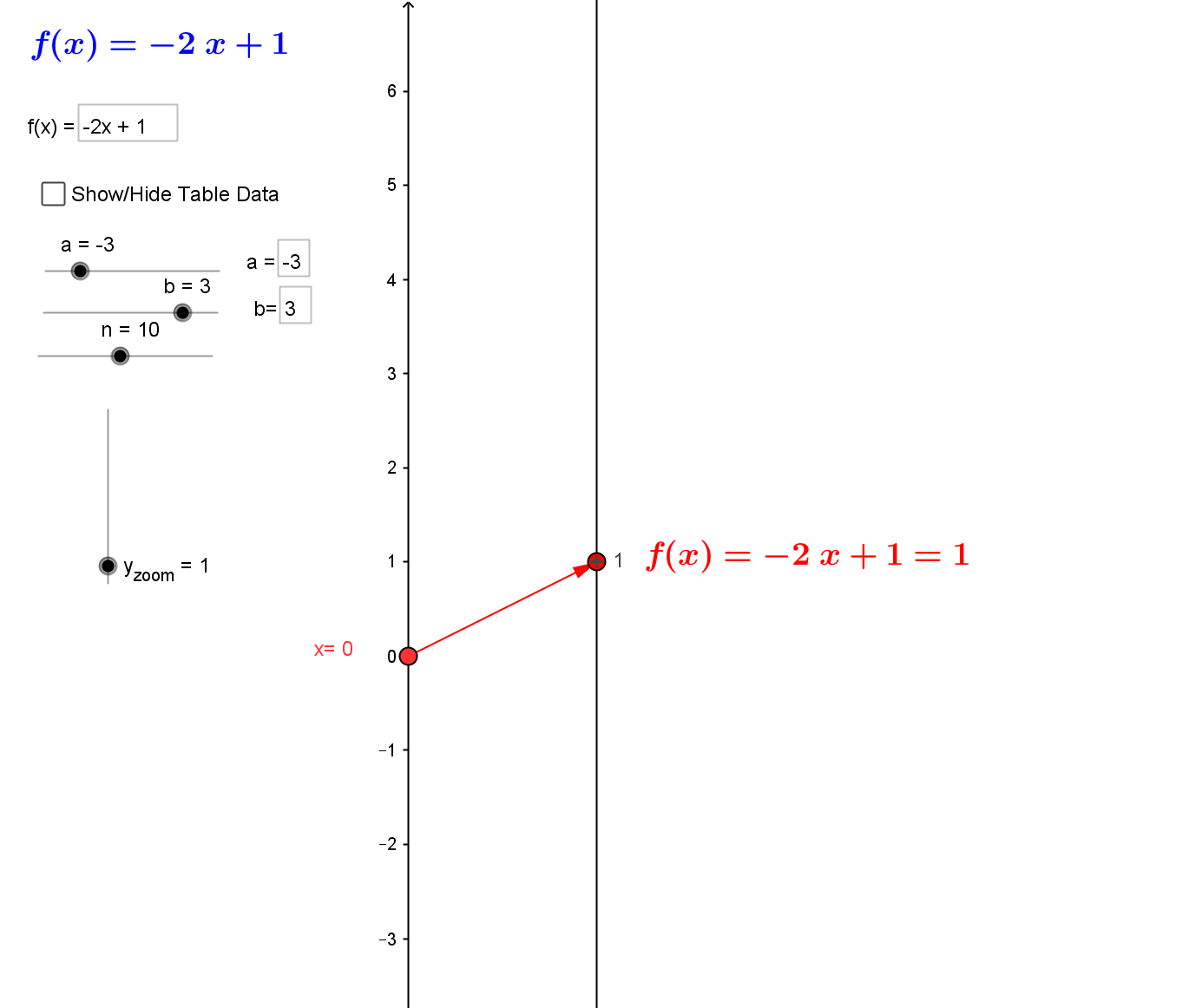x f(x) = -2 x + 1) 3 -5 2.4 -3.8 1.8 -2.6 1.2 -1.4 0.6 -0.2 0 1 -0.6 2.2 -1.2 3.4 -1.8 4.6 -2.4 5.8 -3 7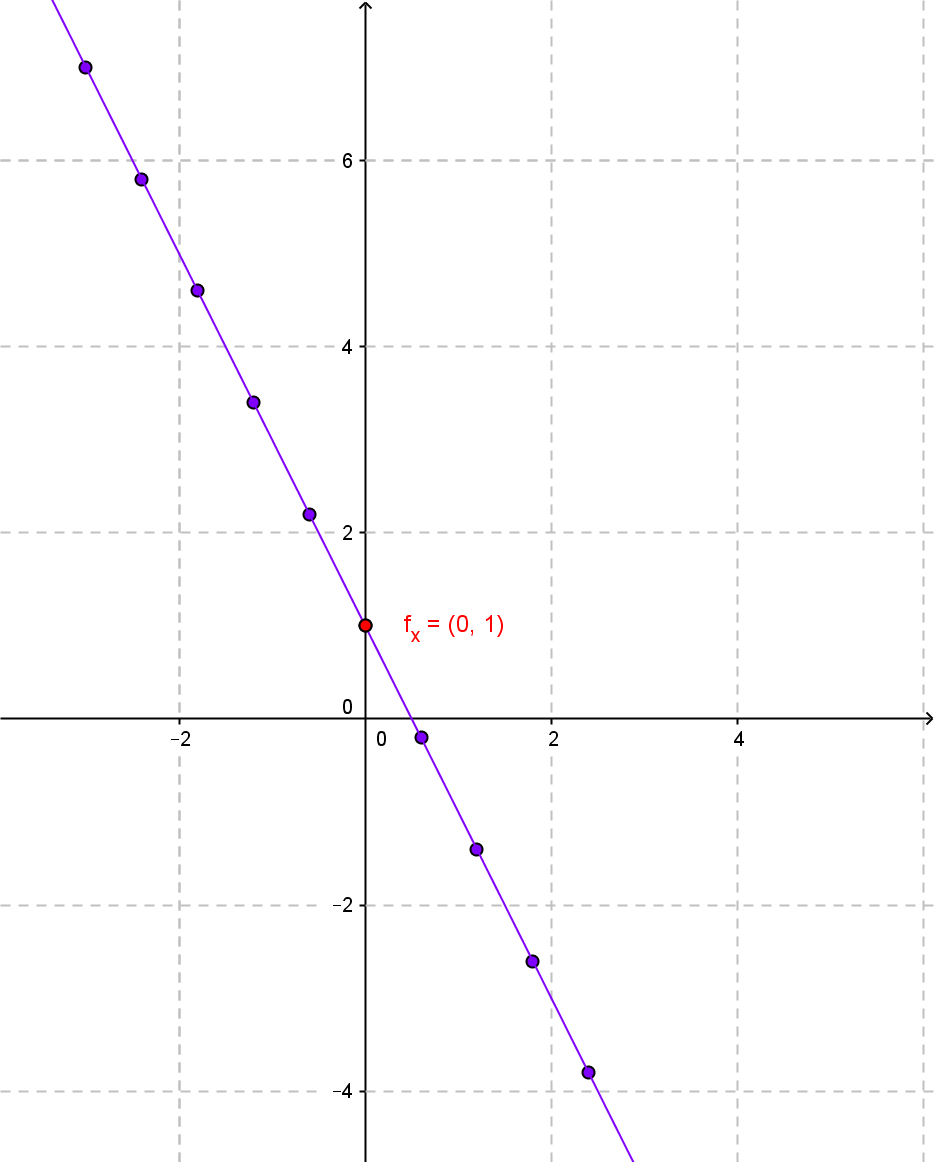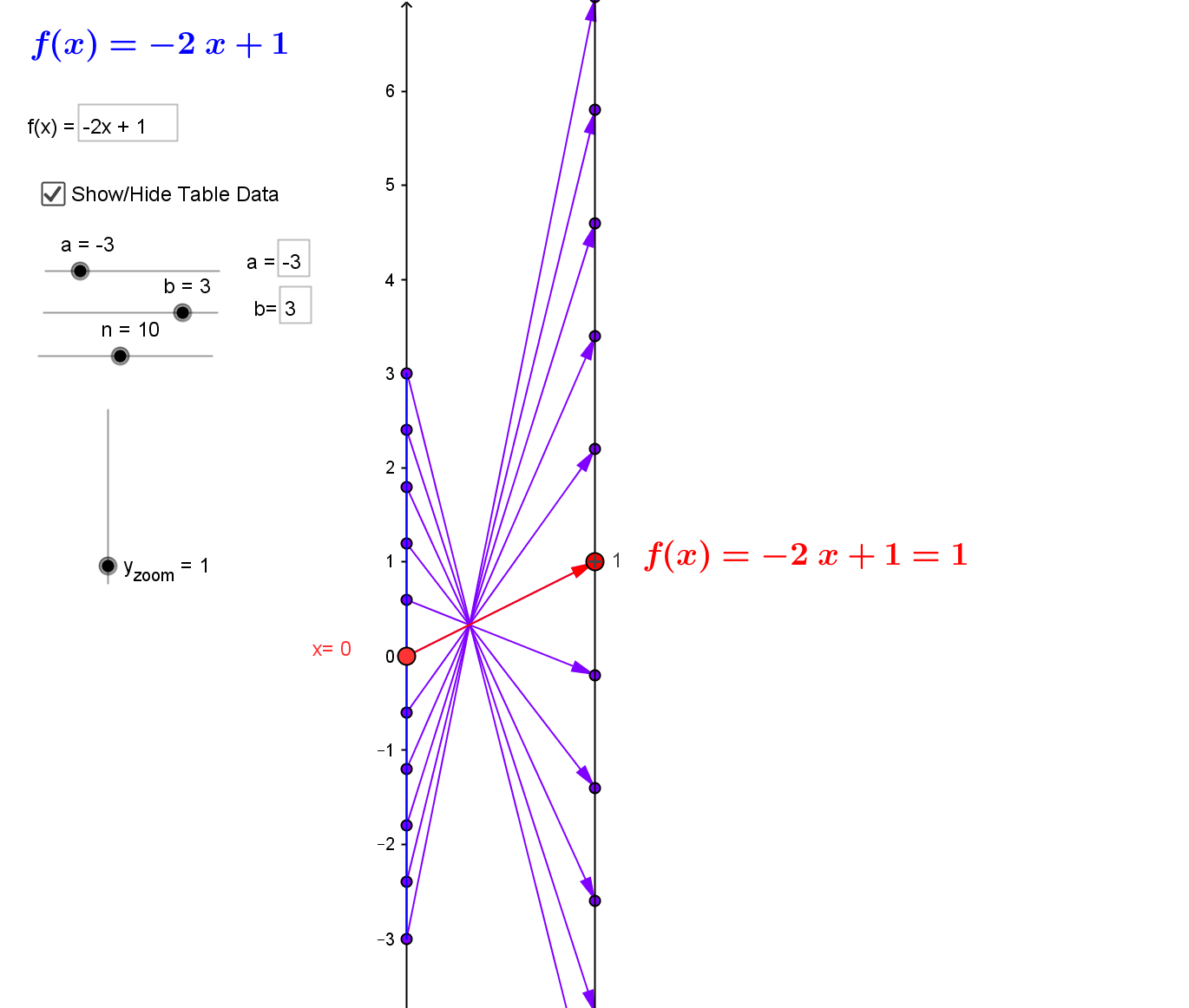2. Linear Functions. ¤
Linear functions are the key to understanding calculus.
[Worksheet 4 and 5.]
Linear functions are traditionally expressed by an equation like :
$f(x)= mx + b$.
Mapping diagrams for linear functions have one simple unifying feature- the focus point, determined by the numbers
$m$ and $b$, denoted here by $[m,b]$
.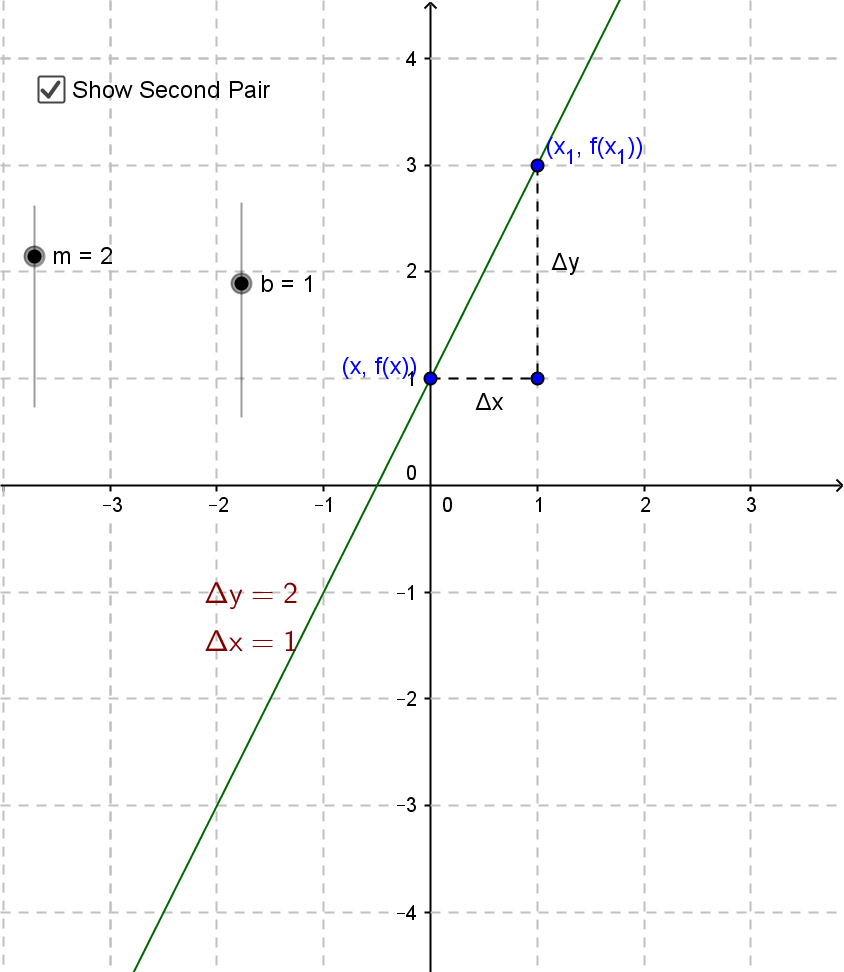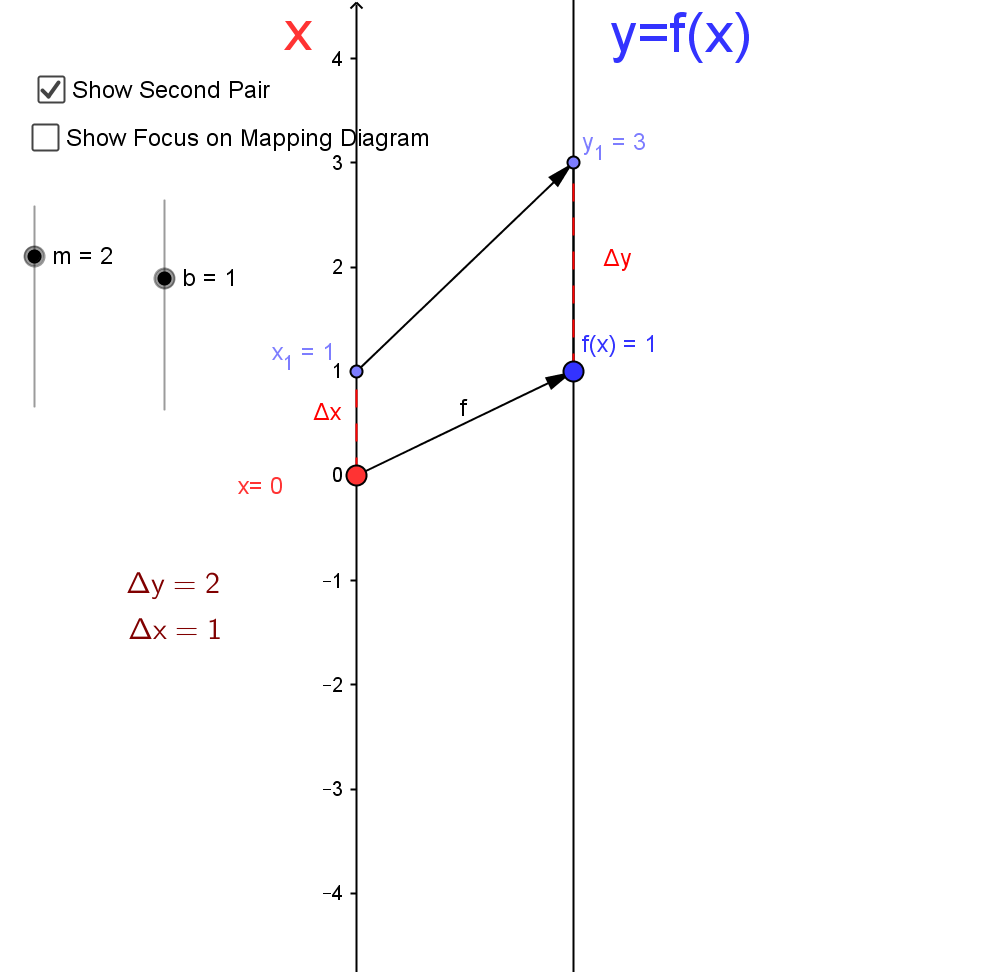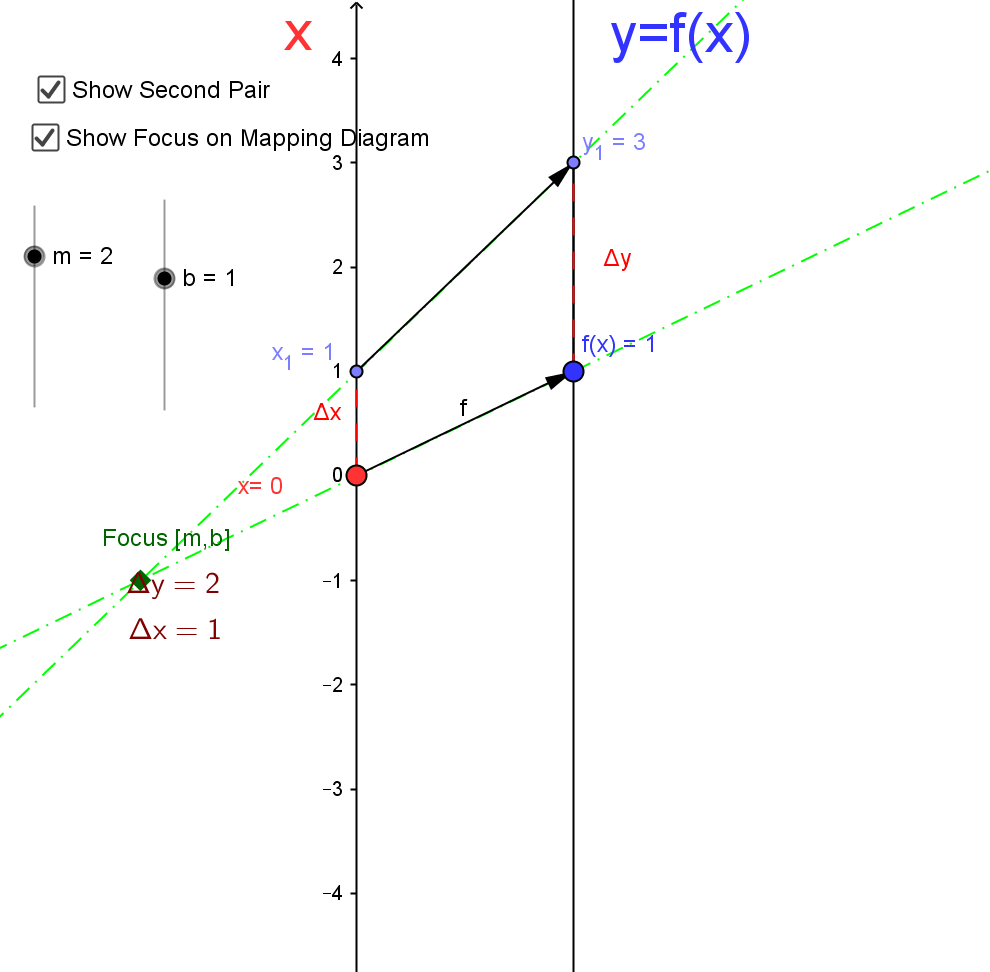Mapping Diagrams and Graphs of Linear Functions
Visualizing linear functions using mapping diagrams and graphs.
This is a Java Applet created using GeoGebra from www.geogebra.org - it looks like you don't have Java installed, please go to www.java.com
Notice how points on the graph pair with arrows and points on the mapping diagram.

3.Limits and The Derivative ¤
Mapping Diagrams Meet Limits and The Derivative
3.1 Limits with Mapping Diagrams and Graphs of Functions
[Worksheet 6 and 7.]
The traditional issue for limits (and continuity) of a function $f$ is whether
$$\lim_{x \rightarrow a}f(x) = L \ and \ \lim_{x \rightarrow a}f(x) = f(a)$$.
The definition is visualized in the following examples.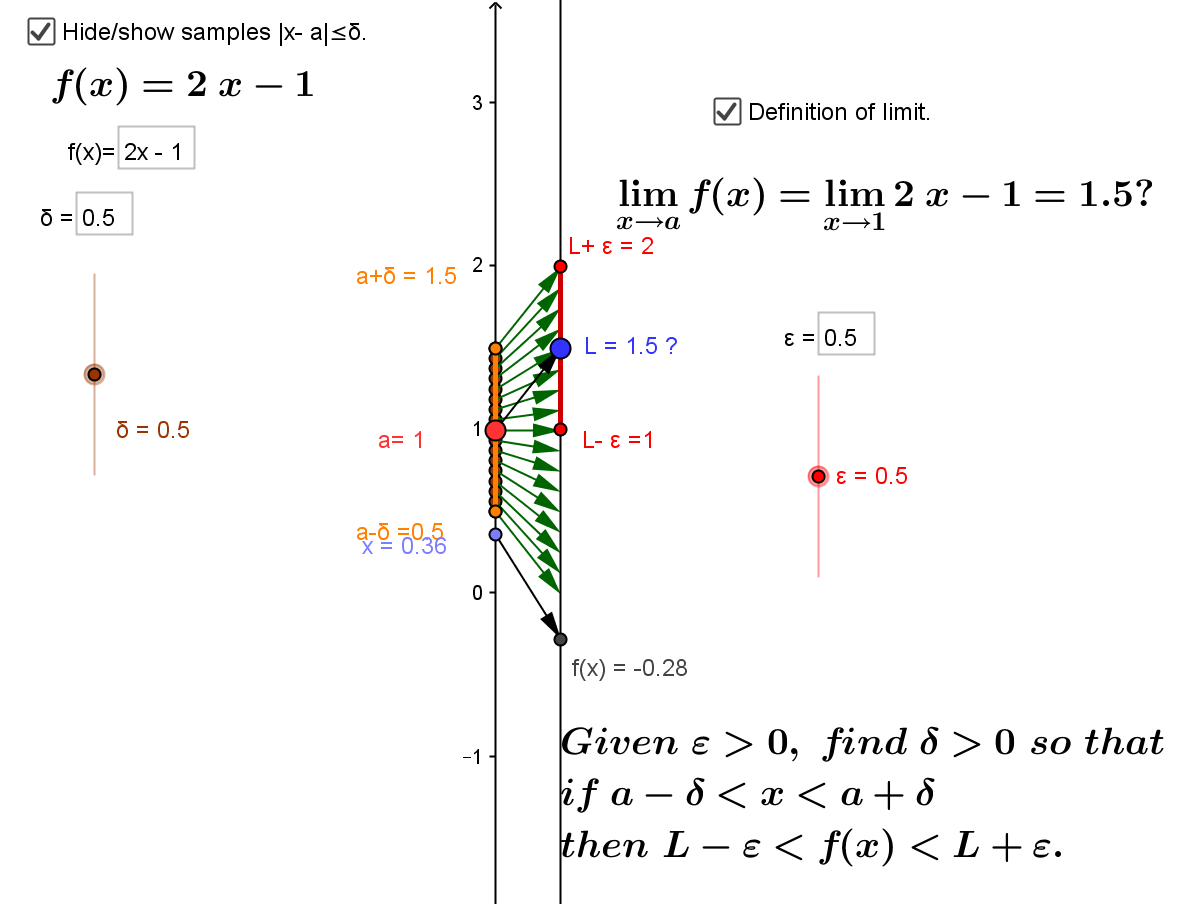Is $$\lim_{x \rightarrow a} 2x - 1 = 1.5?$$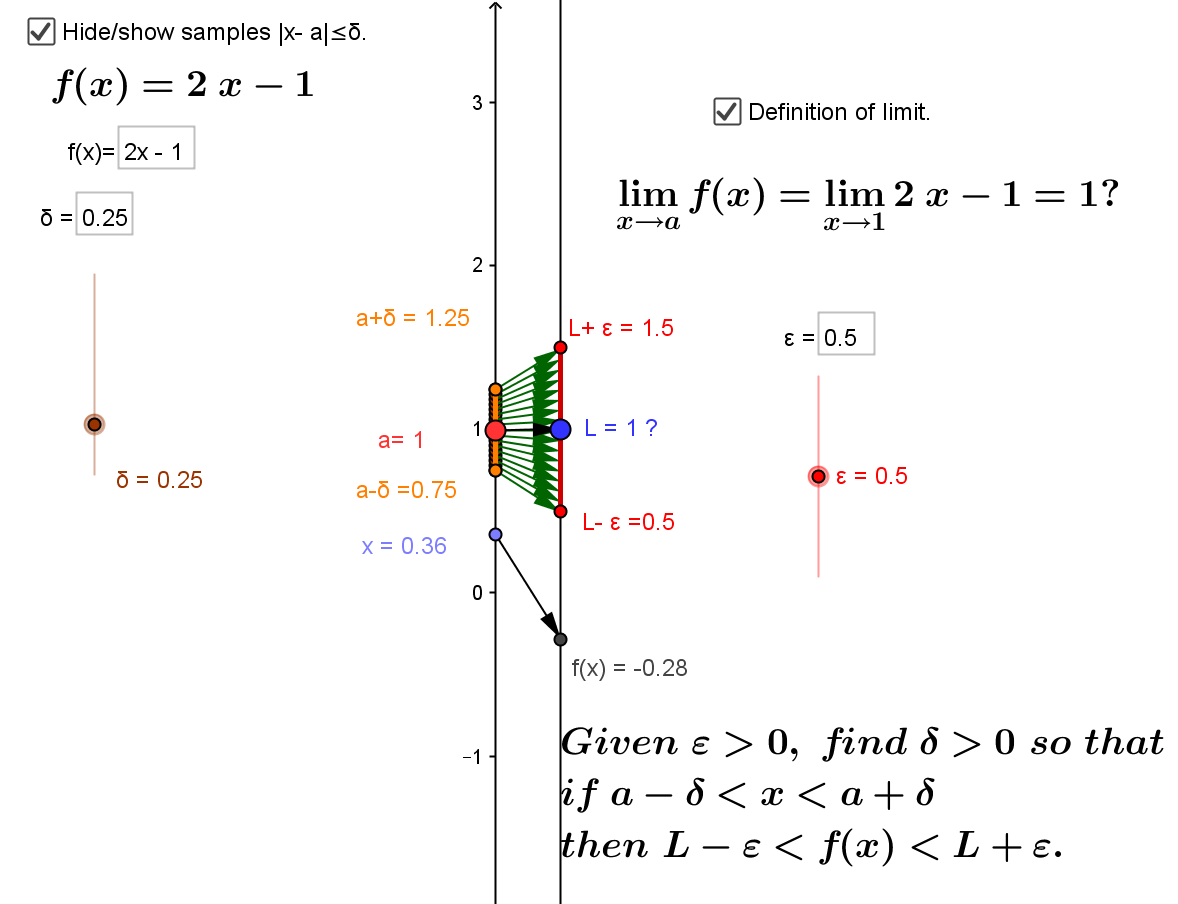Is $$\lim_{x \rightarrow a} 2x - 1 = 1 ?$$

Mapping diagrams and graphs visualize how the definition of a limit (and continuity) works for real functions.
This is a Java Applet created using GeoGebra from www.geogebra.org - it looks like you don't have Java installed, please go to www.java.com
Compare with current GeoGebra Version 5.0.xxx 3D

Notice how points on the graph pair with the points and arrows on the mapping diagram.

3.2 The derivative of $f$ at $a$ is a number, denoted $f'(a)$, defined as a limit of ratios ( average rates or slopes of lines). i.e.,
$$f '(a) = \lim_{x \to a} \frac{f(x)-f(a)}{x-a} \ or\ f'(x) = \lim_{\Delta x \to 0}\frac {f(x+\Delta x) - f(x)}{\Delta x}$$
Four Steps: I. Evaluate: $f(x+\Delta x)$ and $f(x)$
II. Subtract: $\Delta y =f(x+\Delta x) - f(x)$
III. Divide: $\frac {\Delta y}{\Delta x} =\frac {f(x+\Delta x) - f(x)}{\Delta x}$ and simplify if possible.
IV. THINK: As $\Delta x \to 0$, does $\frac {f(x+\Delta x) - f(x)}{\Delta x} \to L$ ? If so, then $L =f'(x)$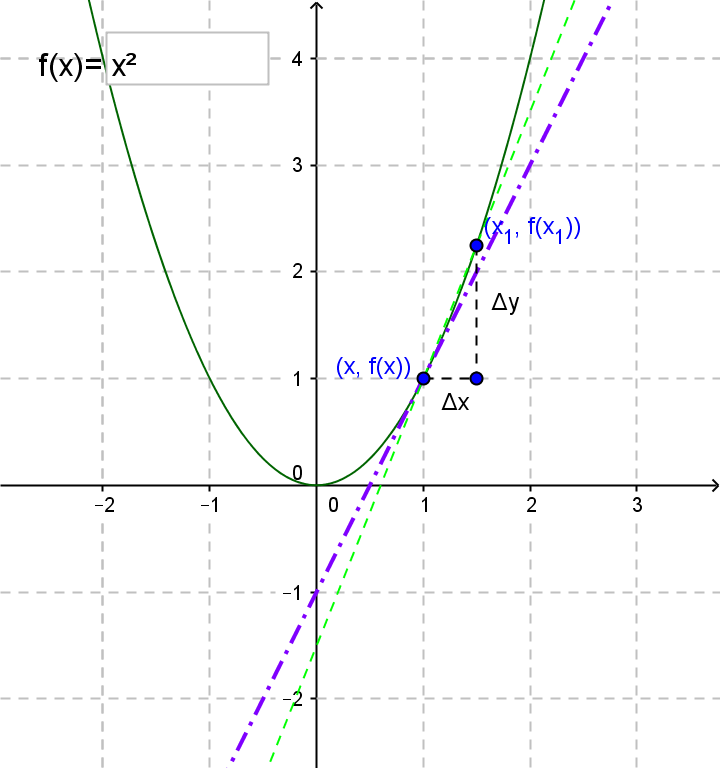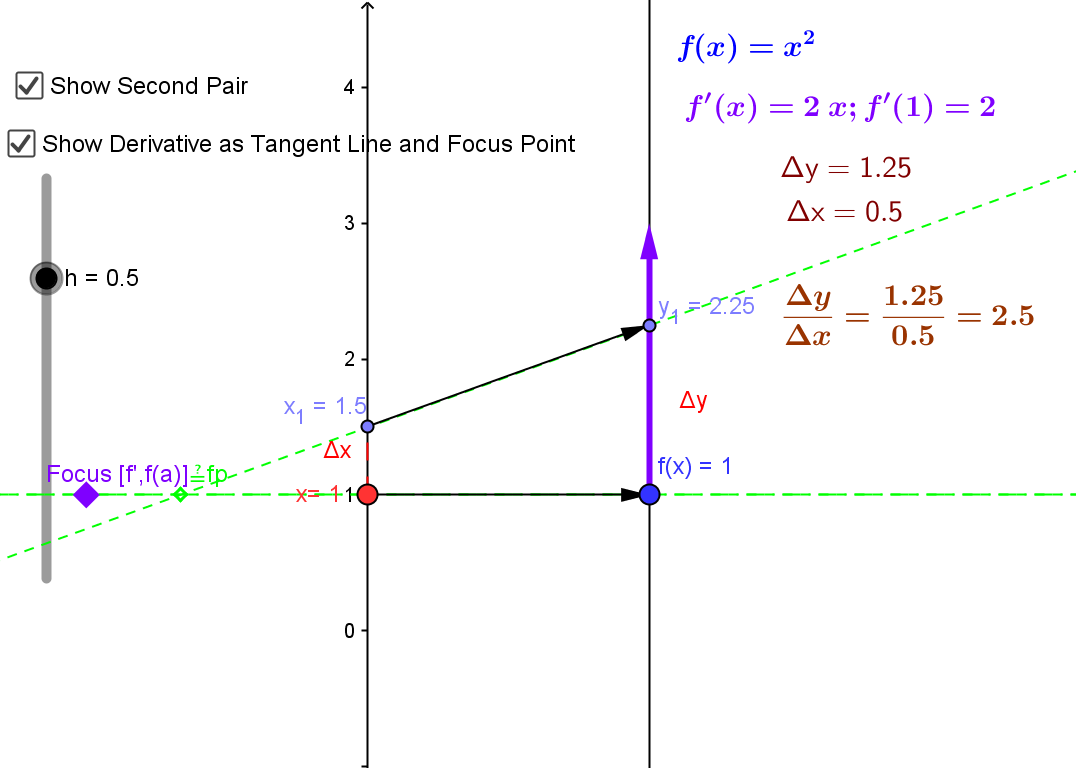The derivative can be visualized using a tangent line on a graph or a focus point and derivative "vector" on a mapping diagram.

The derivative can also be understood as the magnification factor of the best approximating linear function.¤
This is a Java Applet created using GeoGebra from www.geogebra.org - it looks like you don't have Java installed, please go to www.java.com
The derivative is visualized using focus points and derivative "vectors" on a mapping diagram.

In context of Sensible Calculus Text:  SC.I.B [Motivation] Estimating Instantaneous Velocity

3.3 Mapping Diagrams for Composite (Linear) Functions ¤
This is the fundamental concept for the chain rule.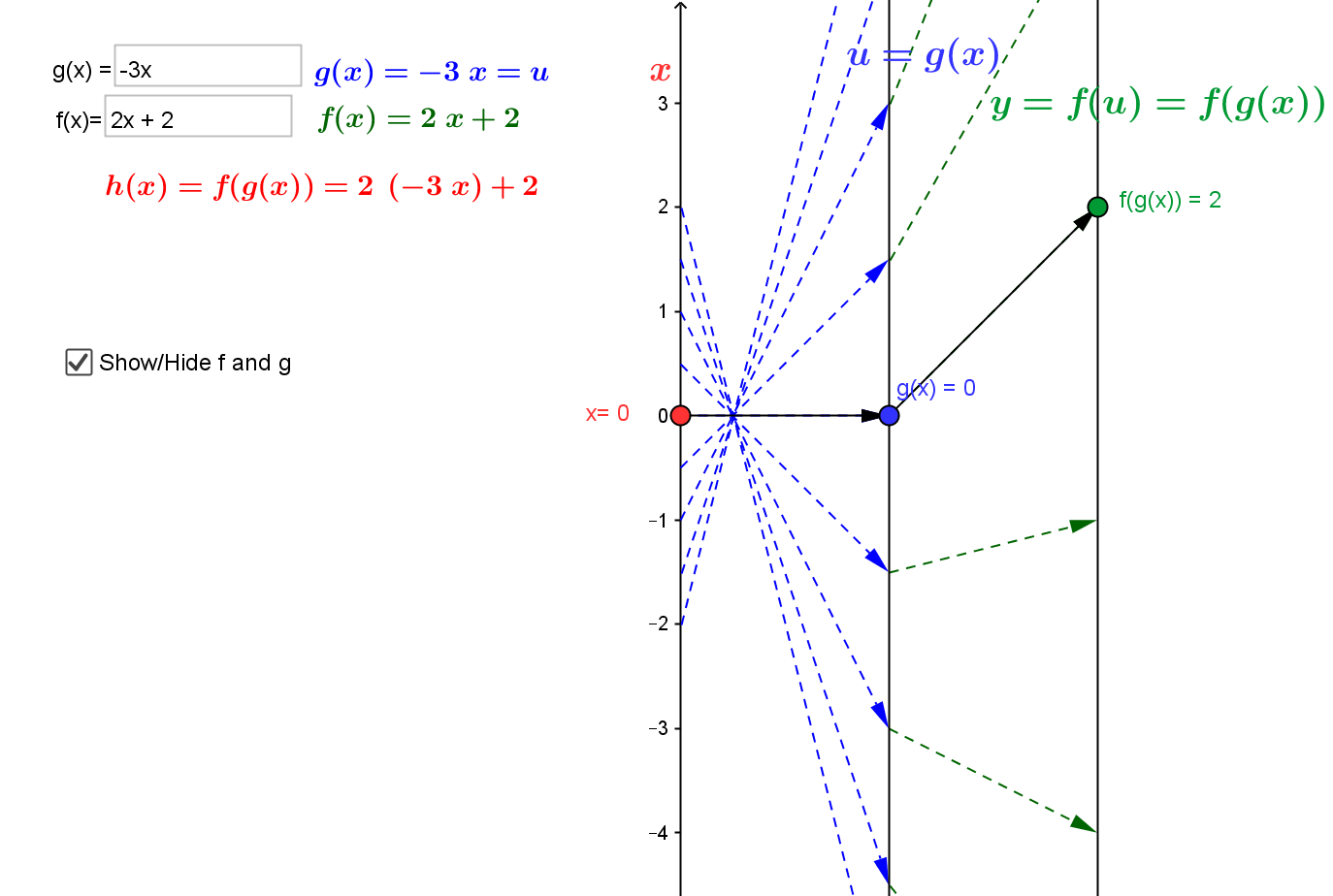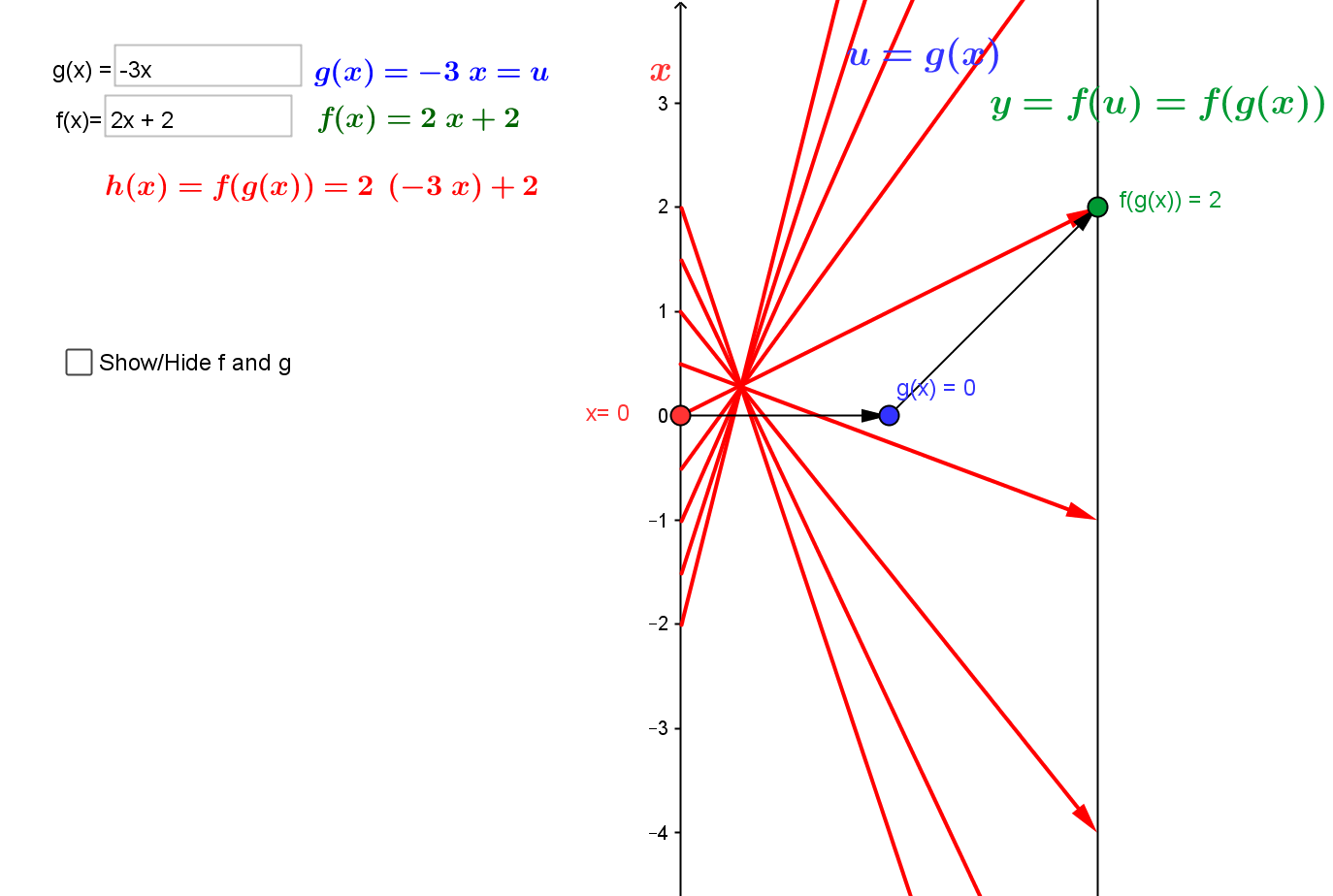Visualizing the composition of linear functions using mapping diagrams.

Composition visualized with GeoGebra.
This is a Java Applet created using GeoGebra from www.geogebra.org - it looks like you don't have Java installed, please go to www.java.com

3.4. Intermediate Value theorem and Newton's Method.
¤
3.4.1. Continuity can be understood by connecting it to the Intermediate Value Theorem (IVT) and solving equations of the form $f(x) = 0$.
IVT: If $f$ is a continuous function on the interval $[a,b]$ and $f(a) \cdot f(b) \gt 0$ then there is a number $c \in (a,b)$ where $f(c) = 0$.
Mapping diagrams provide an alternative visualization for the IVT. They can also be used to visualize a proof of the result using the "bisection method."